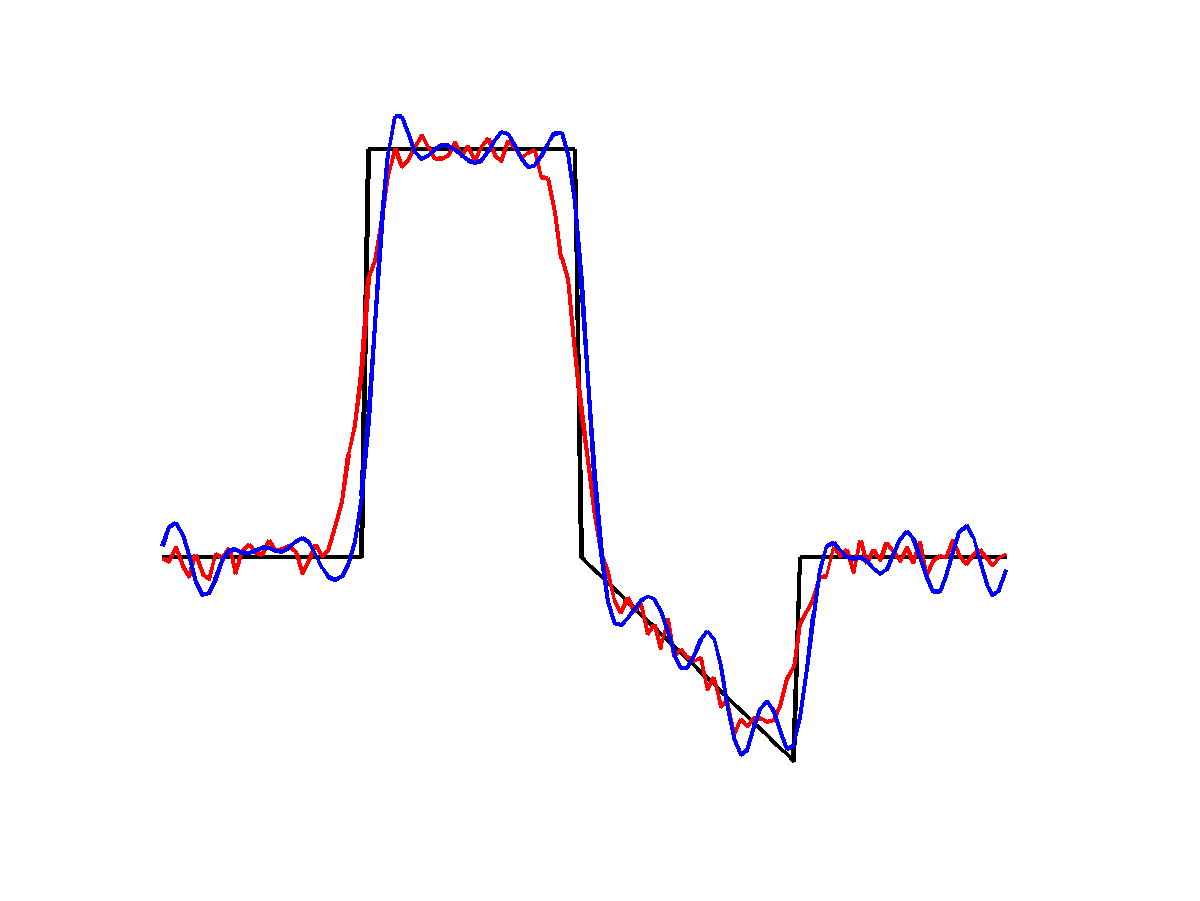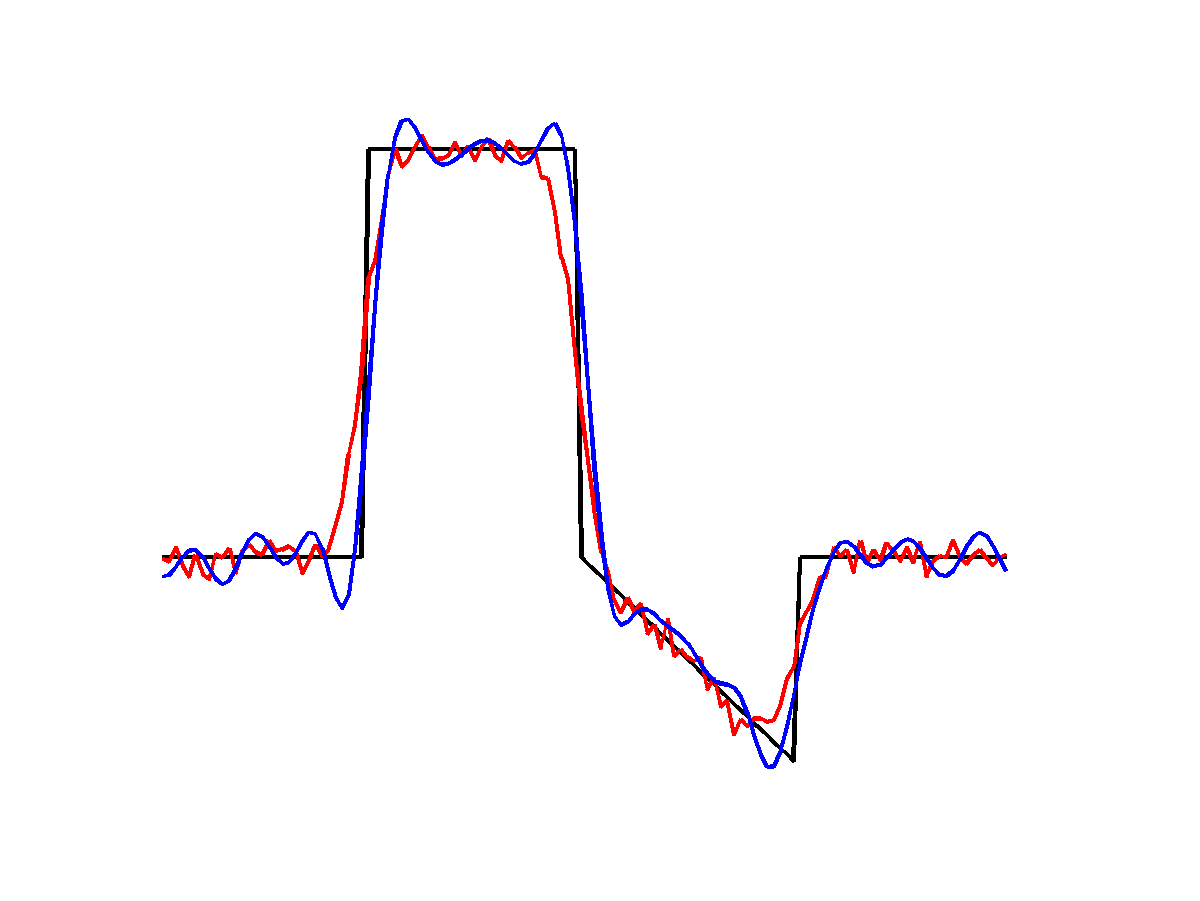## Wednesday, June 18, 2014

### A comparison of some deconvolution priors

Continuing with experiments based on ADMM I implemented and experimented with a few different deconvolution priors.  I chose several $\ell_2$ and $\ell_1$ priors based on sparse gradients, sparse curvature and simply the norm of the solution vector as well as an $\ell_{0.5}$ HyperLaplacian prior.

For all results the ground-truth signal is shown in black, the blurred and corrupted signal in red and the deblurred signal in blue.  As a ground-truth signal I'm using a simple piecewise linear signal with a slanted region, some constant regions and discontinuous jumps.  These features help to show the issues with different regularizers.  The input signal is 128 samples long, blurred by a Gaussian filter with $\sigma=4$ and then corrupted by Gaussian noise with $\sigma=2.5%$ and $\mu=0$.

For the $\ell_2$ results I'm solving for the optimal solution directly in the Fourier domain, while for the $\ell_1$ and $\ell_0.5$ I'm using ADMM, solving the data-subproblem in the Fourier domain and the splitting variable subproblem with shrinkage/proximal operators.  For all problems I've played with the prior weights a bit to try to get good results, but don't claim they are optimal.$\ell_2$ norm of solution vector
First up is the $\ell_2$ norm of the solution.  This does sharpen up the signal somewhat, but has bad ringing artifacts.  These are expected for the $\ell_2$ solution, particularly given the high noise levels in the corrupted signal.  The relatively large blur also leaves lots of freedom for low-frequency ringing.$\ell_2$ norm of solution first derivative
Results for the $\ell_2$-norm of the signal derivatives do a bit better, particularly for the slanted segment, but still show low-frequency ringing.  The same is true for the $\ell_2$-norm of the second derivative, although the ringing is a bit more pronounced in the flat regions:$\ell_2$ norm of solution second derivative
Moving on to the $\ell_1$ priors, the total-variation prior is simply the $1$-norm of the signal first derivatives. It favours piecewise constant solutions which do well on the large flat regions but introduce staircase artifacts for the slanted region:$\ell_1$ norm of solution first derivative
The total variation prior produces a much sharper signal than the $\ell_2$ priors, however for the slanted region it also introduces spurious features in the slanted region that are hard to distinguish from (correct) features elsewhere in the signal.  This occurs because the assumption of the total variation prior is that the input is piecewise constant, which is not true for this signal.  A better assumption is that the solution is piecewise linear, leading to the sparse-curvature prior.  The sparse-curvature assumes that the second derivative of the signal is non-zero in only a few locations:$\ell_1$ norm of solution second derivative
The sparse-curvature prior does much better in the slanted region than the total variation prior and still improves the signal, but the edges are not as sharp at discontinuities.  Often people combine these priors to try to achieve a compromise between the two behaviours.

The final prior is the Hyper-Laplacian prior, which is simply the $\ell_{0.5}$-norm of the signal first derivatives:$\ell_{0.5}$ norm of solution first derivative
The Hyper-Laplacian prior is non-convex, making it difficult to optimize for.  Here it appears that the optimization got stuck: some edges are extremely sharp, however the trailing edge of the high-amplitude constant region is lost entirely, possible because of the slanted region adjacent to it.  I played with the parameters quite a bit but did not get a result better than this.

Matlab example code generating these results is available at my GitHub repository:

https://github.com/jamesgregson/Matlab-deblurring-example